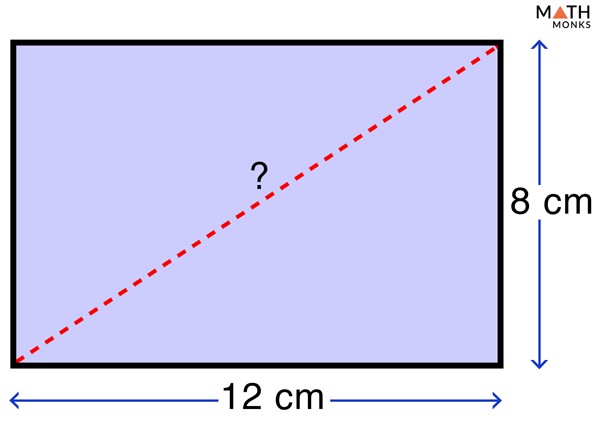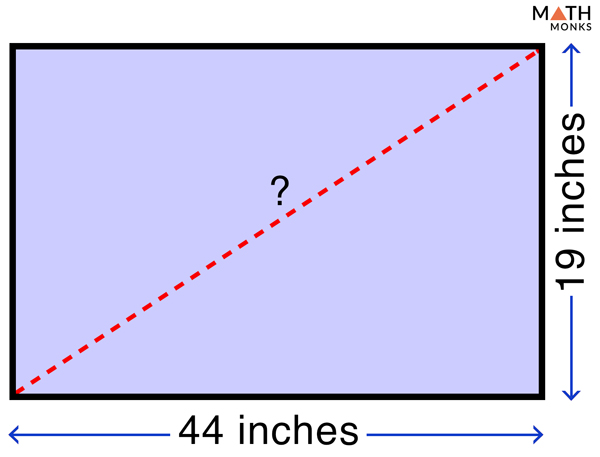# Diagonal of Rectangle

While learning how to find out the area and perimeter of a rectangle, we came across the term diagonal many a times. In this article, we will learn about the diagonal and how to calculate it.

## What is the Diagonal of a Rectangle

The diagonal of a rectangle is the line segments linking opposite vertices or corners of the rectangle.

A rectangle has two diagonals. Each diagonal is a line segment connecting the opposite vertices of a rectangle.

## Properties

1. The 2 diagonals are congruent (equal in length);  here AC and BD are the diagonals & AC = BD
2. The diagonals bisect each other equally; here AC & BD bisect each other
3. Each diagonal divides a rectangle into 2 congruent right-angled triangles; here AC divides ▭ ABCD into 2 ∆ABC and ∆ADC;  BD divides ▭ ABCD into ∆BCD and ∆BAD
4. The diagonals are not perpendicular to each other; here diagonals AC & BD are not perpendicular
5. The adjacent central angles at the point of intersection are not equal, but the opposite central angles are equal; here ∠AOB = ∠COD & ∠BOC = ∠AOD, but ∠AOB  ≠ ∠AOD or ∠BOC, & ∠BOC ≠ ∠COD or ∠AOB

## Formula

The formula to find the diagonal of a rectangle is given below:

### Derivation

Applying Pythagorean theorem in ▭ ABCD, we get,

=> d2 = w2 + l2 , here d = diagonal, l = length, w = width

Taking square root on both sides,

d = √(w2 + l2)

Thus we get the formula to calculate the diagonal of a rectangle.

Let us see how to calculate the diagonals of rectangles by solving some examples.

## Solved ExamplesDetermine the length of the diagonal of a rectangle whose dimensions are 8 cm and 12 cm.

Solution:

As we know,
d = √(w2 + l2), here w = 8 cm, l = 12 cm
= √(82 + 122)
= √(64 + 144)
= √208
≈ 14.42 cmIf the size of a television screen is its diagonal, then find the size of a television whose dimensions are 19 inches and 44 inches.

Solution:

As we know,
d = √(w2 + l2), here w = 19 inches, l = 44 inches
= √(192 + 442)
= 47.93
≈ 48 inches
In the practical world, television sizes are stated as the closest approximate value.

## FAQs

Q1. Do the diagonals of a rectangle bisect each other?

Ans: Yes.

Q2. Do the diagonals of a rectangle bisect the angles?

Ans: No.